Gauge your potential with Aakash BYJU'S JEE Main'23 Mock Test Gauge your potential with Aakash BYJU'S JEE Main'23 Mock Test

# JEE Main 2021 February 26 – Shift 2 Physics Question Paper with Solutions

JEE Main Physics shift 2 question paper along with the answer keys for the exam conducted by NTA on 26th February 2021 is available here. The questions have been solved in a detailed manner by subject experts at BYJU’S so that students can check the correct answers and analyze their performance. Practising these questions will help the JEE aspirants to understand the overall exam pattern and prepare accordingly for upcoming exams. The questions and solutions can be either viewed on this page directly or PDF can be downloaded for offline practice.

### JEE Main 2021 26th February Shift 2 Physics Question Paper

SECTION A

Question 1: A tuning fork A of unknown frequency produces 5 beats/sec with a fork of known frequency 340 Hz. When fork A is filed, the beat frequency decreases to 2 beats/s. What is the frequency of fork A?

1. a. 342 Hz
2. b. 335 Hz
3. c. 338 Hz
4. d. 345 Hz

Solution:

Given

Before filing: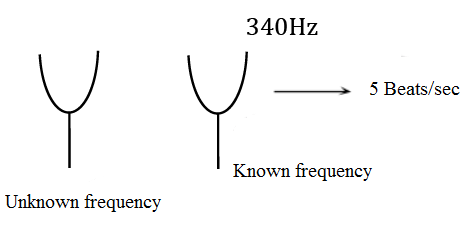So, the answer should be 335 Hz or 345 Hz.

After filing: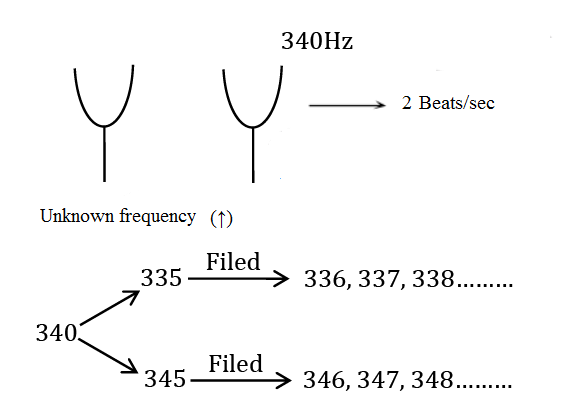After filing beat/sec decreases only in case of 335 Hz.

Question 2: The trajectory of a projectile in a vertical plane is y = αx - βx2, where α and β are constants and x and y, are respectively the horizontal and vertical distances of the projectile from the point of projection. The angle of projection θ and the maximum height attained H are respectively given by

1. a. tan-1α,  α2/4β
2. b. tan-1β,  α2/2β
3. c. tan-1(β/α),  α2
4. d. tan-1α,  4α2

Solution:

Given :

y = αx - βx2 ....(1)

for maximum height, we should find out maximum value of y from equation (1)

so, for maximum value of y

dy/dx = 0

⇒ α -2βx = 0

x = α/2β ....(2)

Now, put value of x from equation (2) in equation (1)

y = α(α/2β) - β(α2/4β2)

⇒ (α2/2β) - (α2/4β)

⇒ α2/4β

So, Hmax = α2/4β ....(3)

As we know maximum height Hmax = u2sin2θ/2g ...(4)

From (3) and (4) u2 = (α2/4β)(2g/sin2θ)

And range (R) = u2×2 sinθ cosθ/g

Note: We can calculate the angle of projection by simply comparing the given equation with equation of trajectory

tanθ = α

⇒ θ = tan-1 (α)

Question 3: A cord is wound around the circumference of a wheel of radius r. The axis of the wheel is horizontal and the moment of inertia about it is I. A weight mg is attached to the cord at the end. The weight falls from rest. After falling through a distance ‘h’, the square of the angular velocity of the wheel will be: (there is no slipping between the wheel and the cord)

1. a. 2gh/(I + mr2)
2. b. 2gh
3. c. 2mgh/(I + 2mr2)
4. d. 2mgh/(I + mr2)

Solution: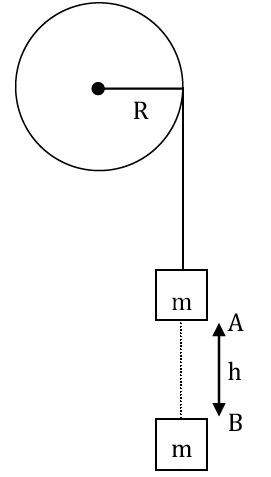Using energy conservation principle between A and B points

mgh = ½ m (ωR)2 + ½ Iω2(∵v = ωr)

2mgh = (mR2 + I) ω2

ω2 = 2mgh/(I + mR2)

Question 4: Find the peak current and resonant frequency of the following circuit (as shown in the figure)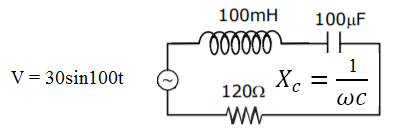1. a. 0.2 A and 100 Hz
2. b. 2 A and 50 Hz
3. c. 2 A and 100 Hz
4. d. 0.2 A and 50 Hz

Solution:

Peak current in series LCR CKT

i = V0/z

⇒ 30/√((XL-XC)2+R2)

i = 30/√((10 - 100)2+120)2

i ⇒ 30/150

⇒1/5 = 0.2Amp.

∴XL = ω×L

⇒ (100) (100 × 10–3) = 10

XC = 1/ω×c

⇒1/100×100×10-6

⇒106/104 = 100

Resonance frequency ω = 1/√(LC)

ω = 1/√(100×10-3×100×10-6) ⇒1/√10-5

∴ω = 2πF

F = (1/2π) (1/√10-5)

⇒ (1/2π)√105

⇒ (100/2π) √10

⇒ 50Hz

Question 5: The incident ray, reflected ray and the outward drawn normal are denoted by the unit vectors

$$\vec{a},\, \vec{b} \; and \; \vec{c}$$
respectively. Then choose the correct relation for these vectors.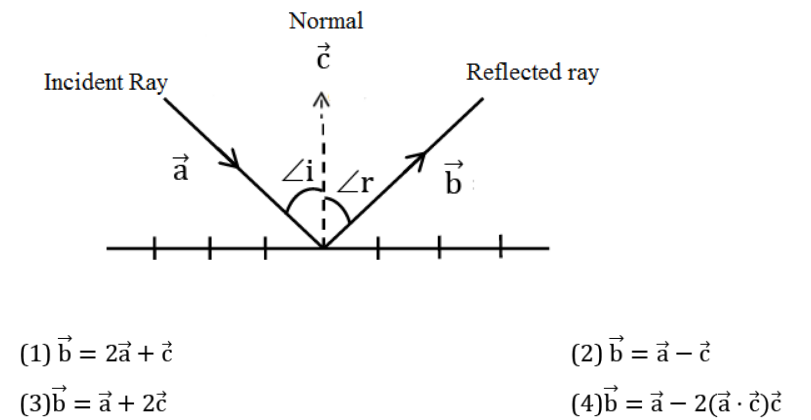Solution: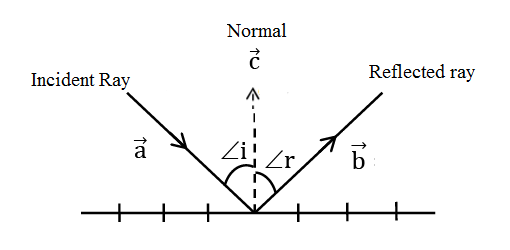We see from the diagram that because of the law of reflection, the component of the unit vector

$$\vec{a}$$
along
$$\vec{b}$$
changes sign on reflection while the component parallel to the mirror remain unchanged.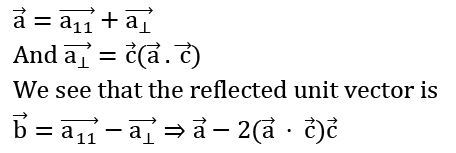Question 6: A radioactive sample is undergoing α decay. At any time t1, its activity is A and at another time t2, the activity is A/5. What is the average lifetime for the sample?

1. a. (t2 - t1 )/ln 5
2. b. ln(t2 + t1) /2
3. c. (t1 - t2)/ln 5
4. d. ln5/(t2 - t1)

Solution:

For activity of radioactive sample :

A =

$$A_{0}e^{-\lambda t_{1}}$$
....(1)

A/5 =

$$A_{0}e^{-\lambda t_{2}}$$
....(2)

From (1)/(2)

5 =

$$e^{-\lambda (t_{1}-t_{2})}$$

ln (5) = (t2 – t1) λ

⇒ λ = ln(5)/t2 - t1

avg. life = 1/λ = (t2 - t1)/ ln(5)

Question 7: A particle executes S.H.M., the graph of velocity as a function of displacement is:

1. a. a circle
2. b. a parabola
3. c. an ellipse
4. d. a helix

Solution:

For a body performing SHM, relation between velocity and displacement

v = ω√(A2 - x2)

Now, square both the sides

v2 = ω2 (A2 - x2)

⇒ v2 = ω2A2 - ω2x2

v2 + ω2x2 = ω2A2

Divide whole equation by ω2A2

v22A2 + ω2x22A2 = ω2A22A2

v2/(ωA)2 + x2/(A)2 = 1

The above equation is similar to the standard equation of an ellipse, so the graph between velocity and displacement will be an ellipse.

Question 8: A scooter accelerates from rest for time t1 at constant rate α1 and then retards at constant rate α2 for time t2 and comes to rest. The correct value of t1/t2 will be:

1. a. (α1 + α2)/α2
2. b. α21
3. c. (α1 + α2)/α1
4. d. α12

Solution:

From given information: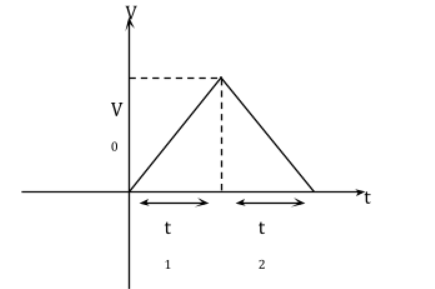For 1st interval

α1 = v0/t1

v0 = α1 t1 …..(1)

For 2nd interval

α2 = v0/t2

v0 = α2 t2 …..(2)

From (1) & (2)

α1 t1 = α2 t2

t1/t2 = α21

Question 9: Draw the output Y in the given combination of gates.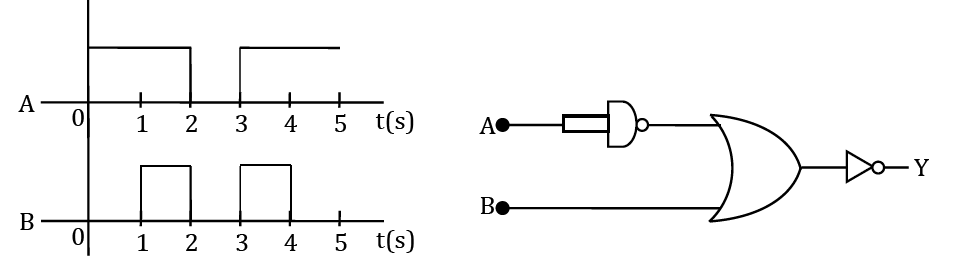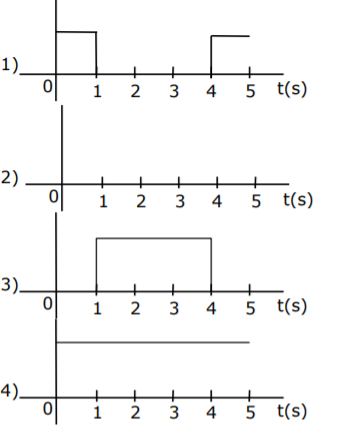Solution: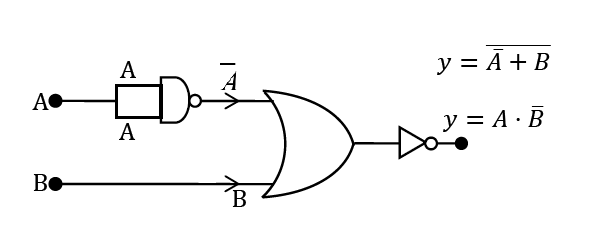Find output expression

$$y=A.\overline{B}$$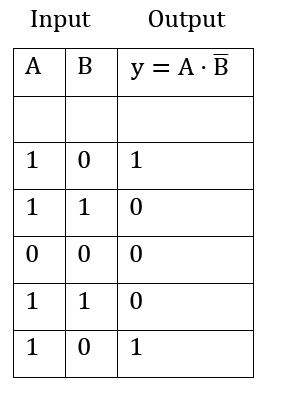Question 10: An inclined plane making an angle of 300 with the horizontal is placed in a uniform horizontal electric field 200  N/C as shown in the figure. A body of mass 1 kg and charge 5 mC is allowed to slide down from rest from a height of 1 m. If the coefficient of friction is 0.2, find the time taken by the body to reach the bottom.

[g = 9.8m/s2, sin 300 = 1/2; cos 300 = √3/2]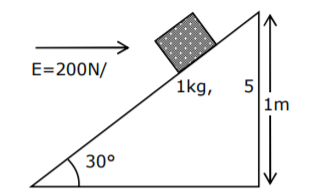1. a. 2.3 s
2. b. 0.46 s
3. c. 1.3 s
4. d. 0.92 s

Solution: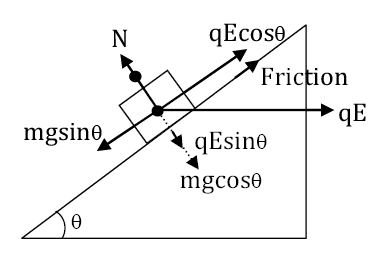Fnet = mg sinθ - (μN + q E cosθ)

Fnet = mg sinθ - μ(mg cosθ + qE sinθ) - qE cosθ

Fnet = 1 × 10 × sin300 – 0.2 (1 × 10 × cos300 + 200 × 5 × 10-3 sin300) - 200 × 5 × 10-3 cos300

F = 2.3 N

a = F/m

⇒ 2.3/1 = 2.3 m/sec2

t = √(2S/a)

⇒√(2×2/2.3) ⇒ 1.3 sec

Question 11: If ‘C’ and ‘V’ represent capacitance and voltage respectively then what are the dimensions of λ where C/V = λ?

1. a. [M-2L-4I3T7]
2. b. [M-2L-3I2T6]
3. c. [M-1L-3I-2T-7]
4. d. [M-3L-4I3T7]

Solution:

∴ V = w/q and C = q/V

Dimension of C/V

⇒ q2/Vw

⇒ (q/V2)× q2

⇒ q3/V2

⇒ I3T3/M2L4T-4

⇒ [M-2L-4T7I3]

Question 12: Given below are two statements: One is labelled as Assertion A and the other is labelled as Reason R.

Assertion A: For a simple microscope, the angular size of the object equals the angular size of the image.

Reason R: Magnification is achieved as the small object can be kept much closer to the eye than 25 cm and hence it subtends at a large angle.

In light of the above statements, choose the most appropriate answer from the options given below:

1. a. Both A and R are true but R is NOT the correct explanation of A
2. b. Both A and R are true and R is the correct explanation of A
3. c. A is true but R is false
4. d. A is false but R is true

Solution: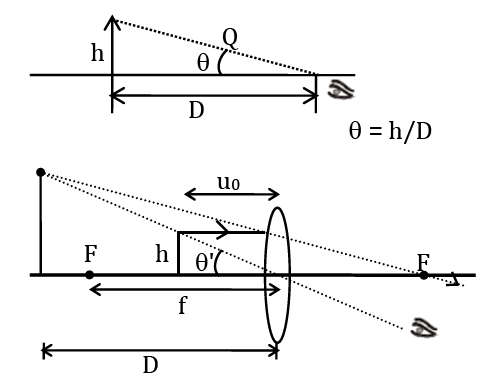Question 13: The recoil speed of a hydrogen atom after it emits a photon in going from n = 5 state to n = 1 state will be:

1. a. 4.17 m/s
2. b. 4.34 m/s
3. c. 219 m/s
4. d. 3.25 m/s

Solution: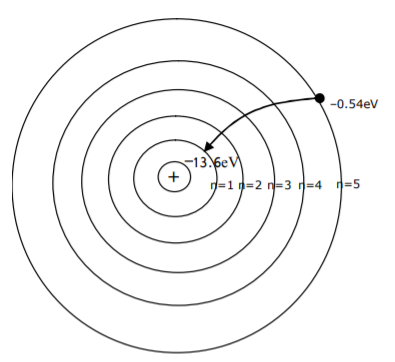Momentum (P) = ΔE/C

⇒ (13.6 - 0.54)eV/3×108

mv = (13.06)×1.6×10-19/3×108

v = (13.06)×1.6×10-19/3×108×1.67×10-27

⇒ 4.17 m/sec

Question 14: Two masses A and B, each of mass M are fixed together by a massless spring. A force acts on mass B as shown in the figure. If mass A starts moving away from mass B with acceleration ‘a’, then the acceleration of mass B will be: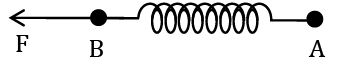1. a. (F + Ma)/M
2. b. (F - Ma)/M
3. c. (Ma - F)/M
4. d. MF/(F + Ma)

Solution: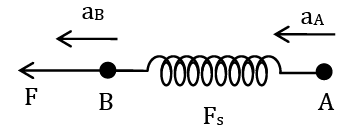For block A

aA = kx/M = a ………..(i)

For block B

aB = (F - kx)/M………(ii)

From equation (i) and (ii)

aB = F/M - kx/M = F/M - a

aB = (F - Ma)/M

Question 15: A wire of 1Ω has a length of 1 m. It is stretched till its length increases by 25%. The percentage change in resistance to the nearest integer is:

1. a. 25%
2. b. 12.5%
3. c. 76%
4. d. 56%

Solution:

∵R ∝  l/A ……….(i)

For stretched or compressed wire

Volume = constant

Area × length = constant

Form equation (i)

R ∝ l2

R1/R2 = l12/l22

⇒ R1/R2 = l2/(1.25l)2

⇒ R2 = 1.5625 R

% increase → 56.235%

Question 16: Given below are two statements:

Statement (1):- A second’s pendulum has a time period of 1 second.

Statement (2):- It takes precisely one second to move between the two extreme positions.

In light of the above statements, choose the correct answer from the options given below.

1. a. Both Statement I and Statement II are false
2. b. Statement I is true but Statement II is false
3. c. Statement I is false but Statement II is true
4. d. Both Statement I and Statement II is true

Solution:

As we know the time period of the second’s pendulum is 2 sec, so statement (1) is incorrect.

Time taken between two extreme points in the second’s pendulum is 1 sec.

The above statement is correct because the time taken by particle performing SHM between two extreme positions is T/2.

Here, T = 2 sec.

So, time = 2/2 = 1 sec

Question 17: An aeroplane with its wings, spread 10 m, is flying at a speed of 180 km/h in a horizontal direction. The total intensity of the magnetic field at that part is 2.5 × 10–4 Wb/m2 and the angle of dip is 600. The emf induced between the tips of the plane wings will be:

1. a. 88.37 mV
2. b. 62.50 mV
3. c. 54.125 mV
4. d. 108.25 mV

Solution: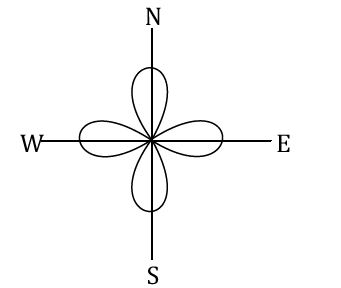E = Bvertical lv

sin 600 = Bv/B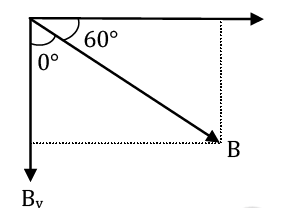√3/2 = Bv/B

Bv = √3B/2

E= (√3/2) Blv

= (√3/2) ×2.5×10-4×10×180×5/18

= (√3/2) ×2.5×5×10-2

= 10.825×10-2

= 108.25 mV

Question 18: The length of the metallic wire is l1 when the tension in it is T1. It is l2 when the tension is T2. The original length of the wire will be:

1. a. (l1 + l2)/2
2. b. (T1l1 - T2l2)/(T2-T1)
3. c. (T2l1 + T1l2)/(T2+T1)
4. d. (T2l1 - T1l2)/(T2-T1)

Solution:

From young’s modulus relation (y = F/A/(Δl/l))

We can write for 1st case

T1/A = y(l1 - l)/l

we can write for 2nd case

T2/A = y(l2 - l)/l

T1/T2 = (l1 - l)/(l2 - l)

T1l2 - T1l = T2l1 - T2l

(T2l1 - T1l2)/(T2 - T1) = l

Question 19: The internal energy (U), pressure (P) and volume (V) of an ideal gas are related as U = 3PV + 4. The gas is:

1. a. polyatomic only
2. b. monoatomic only
3. c. either monoatomic or diatomic
4. d. diatomic only.

Solution:

U = 3 PV + 4

(f/2) PV = 3PV + 4 ∴u = (f/2) nRT

f = 6 + 8/PV ∴Pv = nRT

f>6 ∴ Polyatomic gas.

Question 20: Given below are two statements:

Statement – I: An electric dipole is placed at the centre of a hollow sphere. The flux of the electric field through the sphere is zero but the electric field is not zero anywhere in the sphere.

Statement – II: If R is the radius of a solid metallic sphere and Q be the total charge on it. The electric field at any point on the spherical surface of radius r (< R) is zero but the electric flux passing through this closed spherical surface of radius r is not zero.

In the light of the above statements Choose the correct answer from the option given below:

1. a. Statement I is true but Statement II is false
2. b. Statement I is false but Statement II is true
3. c. Both Statement I and Statement II are true
4. d. Both Statement I and Statement II are false

Solution: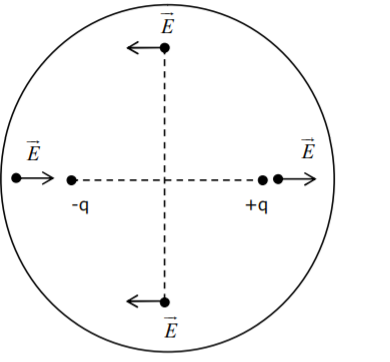Statement 1 → correct: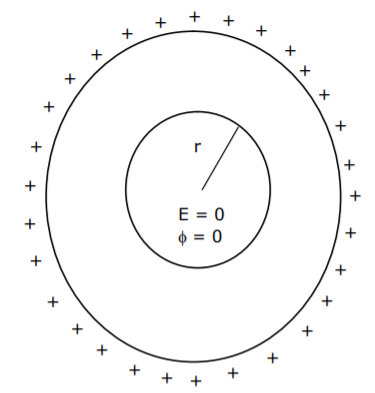Statement 2 → Incorrect:

Statement 2 explanation: The flux through a closed surface due to charges outside it is zero since any field line that enters it, also exits it.

The electric field inside the shell due to a uniformly charged shell in 0, but due to dipole is not 0.

Section - B

Question 1: If the highest frequency modulating a carrier is 5 kHz, then the number of AM broadcast stations accommodated in a 90 kHz bandwidth are ___________.

Solution:

No. of station = Band width/2× Highest Bandwidth

⇒ 90/2×5

⇒ 9

Question 2: 1 mole of a rigid diatomic gas performs a work of Q/5. when heat Q is supplied to it. The molar heat capacity of the gas during this transformation is xR/8. The value of x is _________.

Solution:

From thermodynamics law:

∆Q = ∆U + ∆W …..(1)

Q = nCv∆T + Q/5

Q – Q/5 = 1 × (5/2)R × ∆T

Q = (25/8) RΔT……(2) ∴ Q = ncΔ T

C = (25/8) R

Given C = xR/8

x = 25

Question 3: A particle executes S.H.M with amplitude ‘a’ and time period T. The displacement of the particle from the mean position when its speed is half of the maximum speed is √xa/2. The value of x is ________

Solution:

For a particle executing S.H.M

V = ω√(a2 - x2)

Given V = Vmax/2 ⇒ aω/2

a2ω2/4 = ω2a2 - ω2x2

x = (√3/2)a

Question 4: Two stream of photons, possessing energies equal to twice and ten times the work function of metal are incident on the metal surface successively. The value of the ratio of maximum velocities of the photoelectrons emitted in the two respective cases is x : 3. The value of x is ________.

Solution:

According to Einstein’s law of PE effect Emax = E - ϕ

E1 = 2 ϕ, k1 = ϕ

E2 = 10 ϕ, k2 = 9 ϕ

∴V∝√k (k = ½ mv2)

v1/v2 = √(1/9)

⇒1/3 = x/y

x = 1

Question 5: A point source of light S, placed at a distance 60 cm in front of the centre of the plane mirror of width 50 cm, hangs vertically on a wall. A man walks in front of the mirror along a line parallel to the mirror at a distance 1.2 m from it (see in the figure). The distance between the extreme points where he can see the image of the light source in the mirror is ____cm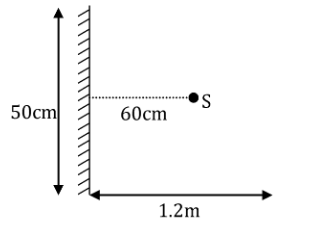Solution:

From similar triangle IMP and IQR

QR/25 = 180/60

⇒ QR = 75 cm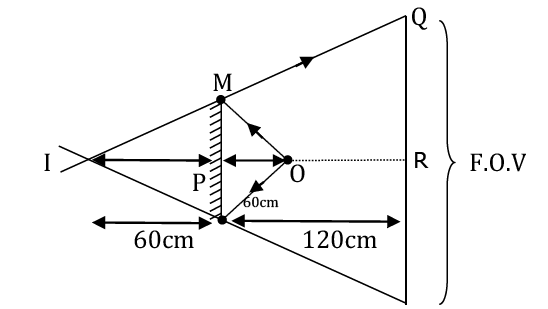Field of view = 2 × 75 ⇒ 150 cm

Question 6: The Zener diode has a VZ = 30 V. The current passing through the diode for the following circuit is______mA.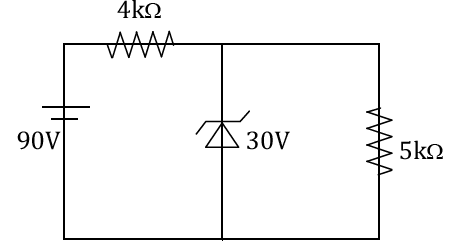Solution: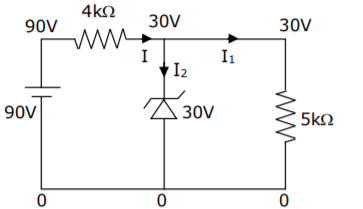I = (90 - 30)/4 = 15 mA

II = 30/5KΩ = 6mA

Using K.C.L.

I2 = 15 mA - 6 mA

= 9 mA

Question 7: In the reported figure of the earth, the value of acceleration due to gravity is the same at point A and C but it is smaller than that of its value at point B (surface of the earth). The value of OA : AB will be x : 5. The value of x is _______________.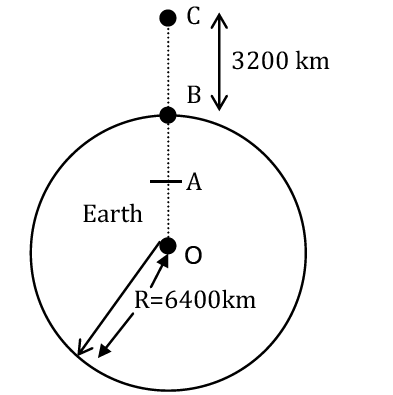Solution:

g = gravitational field of earth

For inside point Eg = GMr/R3

For outside point Eg = GM/r2

GM/(3R/2)2 = GMr/R3

OA = 4R/9 = r

AB = R - 4R/9 = 5R/9

OA : AB

4R/9 : 5R/9

⇒ 4:5 = x:y

⇒ x = 4

Question 8: 27 similar drops of mercury are maintained at 10 V each. All these spherical drops combine into a single big drop. The potential energy of the bigger drop is _____ times that of a smaller drop.

Solution:

The self - energy of the sphere (conducting)

U = kq2/2r

For small drop →Ui = kq2/2r …….(1)

After combine small drops volume remains same as bigger drop

∴ (4/3) πr3×n = (4/3)πR3

R = (n)1/3r ……….(2)

For large drop → Uf = k(nq)2/2×3R ……….(3)

From equation (1), (2), (3)

Uf/Ui = (n)5/3

⇒ (27)5/3

⇒ 243

Question 9: The volume V of a given mass of monatomic gas changes with temperature T according to the relation V = KT2/3. The work done when temperature changes by 90 K will be xR. The value of x is ____. [R = universal gas constant]

Solution:

Given: V = KT2/3

V3/2 = (K)3/2 T

TV–3/2 = const. ……(1)

and TV γ-1 = const.……(2)

From (1) & (2)

-3/2 = γ-1

γ = -1/2

Work done (w) = nRΔT/(γ-1)

W = 1×R×90/-½ -1

|W| = 60R

x = 60

Question 10: Time period of a simple pendulum is T. The time taken to complete ⅝ oscillations starting from mean position is (α/12)T. The value of α is ___________.

Solution: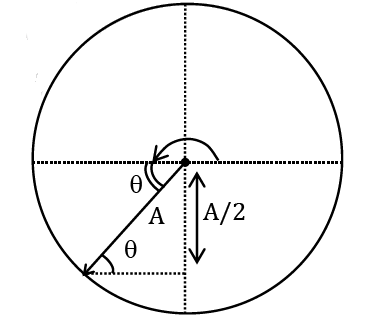th of oscillation = (½ +⅛) th of oscillation

π + θ = ω t

π + π/6 = (2π/T)t

7π/6 = (2π/T)t

t = 7T/12

### JEE Main 2021 Physics Paper February 26 Shift 2